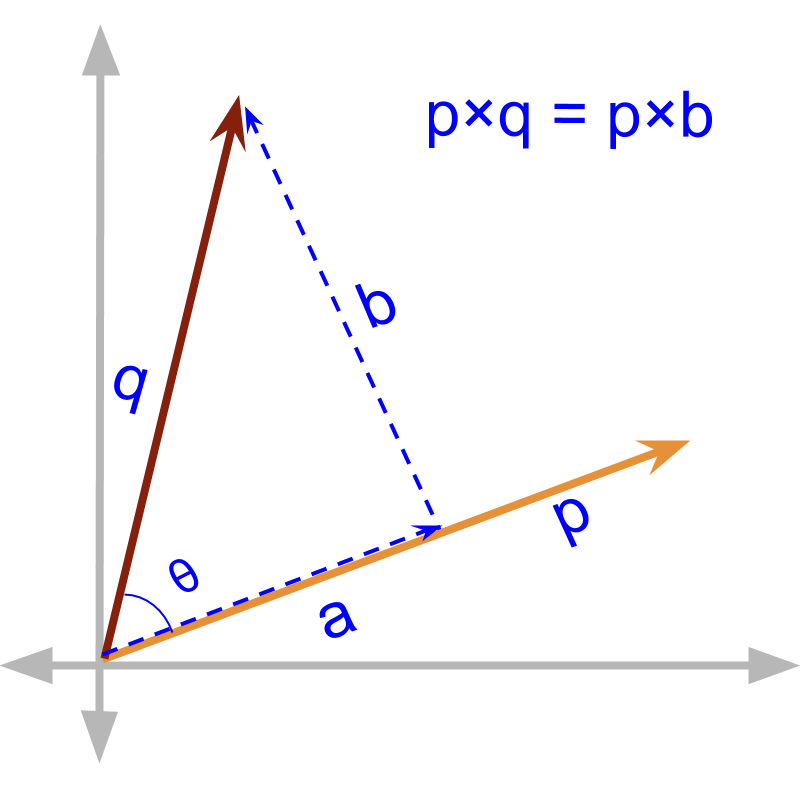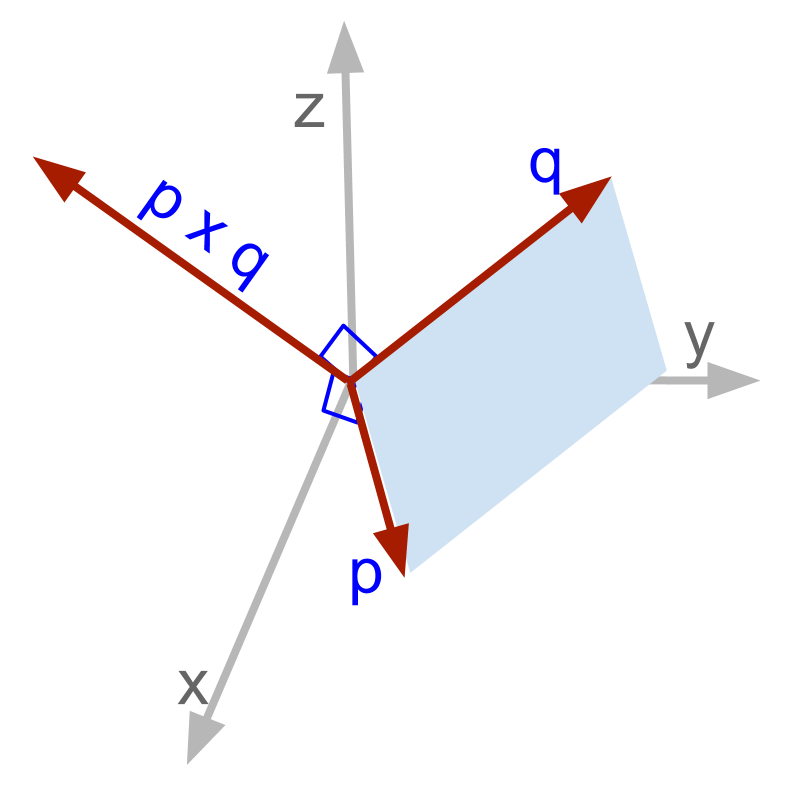maths > vector-algebra

Cross Product: Component Form

what you'll learn...

Overview

Vector Cross Product: Component Form

$\stackrel{\to }{p}×\stackrel{\to }{q}=|\begin{array}{ccc}i& j& k\\ {p}_{x}& {p}_{y}& {p}_{z}\\ {q}_{x}& {q}_{y}& {q}_{z}\end{array}|$$\vec{p} \times \vec{q} = | \left(i , j , k\right) , \left({p}_{x} , {p}_{y} , {p}_{z}\right) , \left({q}_{x} , {q}_{y} , {q}_{z}\right) |$

$=\left({p}_{y}{q}_{z}-{p}_{z}{q}_{y}\right)i$$= \left({p}_{y} {q}_{z} - {p}_{z} {q}_{y}\right) i$ $+\left({p}_{z}{q}_{x}-{p}_{x}{q}_{z}\right)j$$+ \left({p}_{z} {q}_{x} - {p}_{x} {q}_{z}\right) j$ $+\left({p}_{x}{q}_{y}-{p}_{y}{q}_{x}\right)k$$+ \left({p}_{x} {q}_{y} - {p}_{y} {q}_{x}\right) k$

in component formGiven $\stackrel{\to }{p}={p}_{x}i+{p}_{y}j+{p}_{z}k$$\vec{p} = {p}_{x} i + {p}_{y} j + {p}_{z} k$ and $\stackrel{\to }{q}={q}_{x}i+{q}_{y}j+{q}_{z}k$$\vec{q} = {q}_{x} i + {q}_{y} j + {q}_{z} k$ and $|\stackrel{\to }{p}×\stackrel{\to }{q}|= |p||q|\mathrm{sin}\theta$
How to find $\mathrm{sin}\theta$$\sin \theta$, or the cross product in the component form?

Component form of vector cross product : geometrical proof

$|\stackrel{\to }{p}×\stackrel{\to }{q}|$$| \vec{p} \times \vec{q} |$

Substitute the following
${|\stackrel{\to }{p}|}^{2}=\left({p}_{x}^{2}+{p}_{y}^{2}+{p}_{z}^{2}\right)$$| \vec{p} {|}^{2} = \left({p}_{x}^{2} + {p}_{y}^{2} + {p}_{z}^{2}\right)$
${|\stackrel{\to }{q}|}^{2}=\left({q}_{x}^{2}+{q}_{y}^{2}+{q}_{z}^{2}\right)$$| \vec{q} {|}^{2} = \left({q}_{x}^{2} + {q}_{y}^{2} + {q}_{z}^{2}\right)$
${|\stackrel{\to }{p}\cdot \stackrel{\to }{q}|}^{2}={\left({p}_{x}{q}_{x}+{p}_{y}{q}_{y}+{p}_{z}{q}_{z}\right)}^{2}$$| \vec{p} \cdot \vec{q} {|}^{2} = {\left({p}_{x} {q}_{x} + {p}_{y} {q}_{y} + {p}_{z} {q}_{z}\right)}^{2}$
and after some algebraic rearrangement, the following is arrived at

$|\stackrel{\to }{p}×\stackrel{\to }{q}|$$| \vec{p} \times \vec{q} |$

$\quad \quad \quad \quad \overline{+ {\left({p}_{z} {q}_{x} - {p}_{x} {q}_{z}\right)}^{2}}$
$\quad \quad \quad \quad \overline{+ {\left({p}_{x} {q}_{y} - {p}_{y} {q}_{x}\right)}^{2}}$

$\quad \quad \quad \quad + \left({p}_{z} {q}_{x} - {p}_{x} {q}_{z}\right) j$
$\quad \quad \quad \quad + \left({p}_{x} {q}_{y} - {p}_{y} {q}_{x}\right) k |$

It is proven that
$\stackrel{\to }{p}×\stackrel{\to }{q}$$\vec{p} \times \vec{q}$
$\quad \quad = \left({p}_{y} {q}_{z} - {p}_{z} {q}_{y}\right) i$
$\quad \quad \quad \quad + \left({p}_{z} {q}_{x} - {p}_{x} {q}_{z}\right) j$
$\quad \quad \quad \quad + \left({p}_{x} {q}_{y} - {p}_{y} {q}_{x}\right) k$

The same can be written in the determinant form
$\stackrel{\to }{p}×\stackrel{\to }{q}=|\begin{array}{ccc}i& j& k\\ {p}_{x}& {p}_{y}& {p}_{z}\\ {q}_{x}& {q}_{y}& {q}_{z}\end{array}|$$\vec{p} \times \vec{q} = | \left(i , j , k\right) , \left({p}_{x} , {p}_{y} , {p}_{z}\right) , \left({q}_{x} , {q}_{y} , {q}_{z}\right) |$

easier proof

The proof given above is complex. A simpler proof, that a student can easily derive, is given below.

Bilinear Property: For any vector $\stackrel{\to }{p},\stackrel{\to }{q},\stackrel{\to }{r}\in V$$\vec{p} , \vec{q} , \vec{r} \in \mathbb{V}$ and $\lambda \in ℝ$$\lambda \in \mathbb{R}$
$\left(\lambda \stackrel{\to }{p}+\stackrel{\to }{q}\right)×\stackrel{\to }{r}$$\left(\lambda \vec{p} + \vec{q}\right) \times \vec{r}$$=\lambda \left(\stackrel{\to }{p}×\stackrel{\to }{r}\right)+\left(\stackrel{\to }{q}×\stackrel{\to }{r}\right)$$= \lambda \left(\vec{p} \times \vec{r}\right) + \left(\vec{q} \times \vec{r}\right)$
This is explained and proven in properties of the cross product. For now, consider this to be true.

A vector $\stackrel{\to }{p}={p}_{x}i+{p}_{y}j+{p}_{z}k$$\vec{p} = {p}_{x} i + {p}_{y} j + {p}_{z} k$ is sum of scalar multiple of vectors. $i,j,k$$i , j , k$ are unit vectors, and the scalar multiples are ${p}_{x},{p}_{y},{p}_{z}$${p}_{x} , {p}_{y} , {p}_{z}$.

The same applies to $\stackrel{\to }{q}={q}_{x}i+{q}_{y}j+{q}_{z}k$$\vec{q} = {q}_{x} i + {q}_{y} j + {q}_{z} k$ -- sum of multiple of vectors.

Proof for component form of vector cross product using bilinear property of cross product.

$\stackrel{\to }{p}×\stackrel{\to }{q}$$\vec{p} \times \vec{q}$
$\quad \quad = \left({p}_{x} i + {p}_{y} j + {p}_{z} k\right) \times$
$\quad \quad \quad \quad \left({q}_{x} i + {q}_{y} j + {q}_{z} k\right)$
Applying bilinear property of cross product
$\quad \quad = {p}_{x} i \times \left({q}_{x} i + {q}_{y} j + {q}_{z} k\right)$
$\quad \quad \quad \quad + {p}_{y} j \times \left({q}_{x} i + {q}_{y} j + {q}_{z} k\right)$
$\quad \quad \quad \quad + {p}_{z} k \times \left({q}_{x} i + {q}_{y} j + {q}_{z} k\right)$
Applying $i×i=0$$i \times i = 0$, $j×j=0$$j \times j = 0$, $k×k=0$$k \times k = 0$
$i×j=k$$i \times j = k$, $j×k=i$$j \times k = i$, $k×i=j$$k \times i = j$
$j×i=-k$$j \times i = - k$, $k×j=-i$$k \times j = - i$, $i×k=-j$$i \times k = - j$

After algebraic rearrangement, we get
$\stackrel{\to }{p}×\stackrel{\to }{q}$$\vec{p} \times \vec{q}$

$\quad \quad \quad \quad + \left({p}_{z} {q}_{x} - {p}_{x} {q}_{z}\right) j$
$\quad \quad \quad \quad + \left({p}_{x} {q}_{y} - {p}_{y} {q}_{x}\right) k$

summaryVector Cross Product: for vectors $\stackrel{\to }{p},\stackrel{\to }{q}\in {ℝ}^{3}$$\vec{p} , \vec{q} \in {\mathbb{R}}^{3}$
$\stackrel{\to }{p}×\stackrel{\to }{q}=|\begin{array}{ccc}i& j& k\\ {p}_{x}& {p}_{y}& {p}_{z}\\ {q}_{x}& {q}_{y}& {q}_{z}\end{array}|$$\vec{p} \times \vec{q} = | \left(i , j , k\right) , \left({p}_{x} , {p}_{y} , {p}_{z}\right) , \left({q}_{x} , {q}_{y} , {q}_{z}\right) |$

Vector Cross Product between two vectors in component form is defined as a vector from a determinant.

Outline